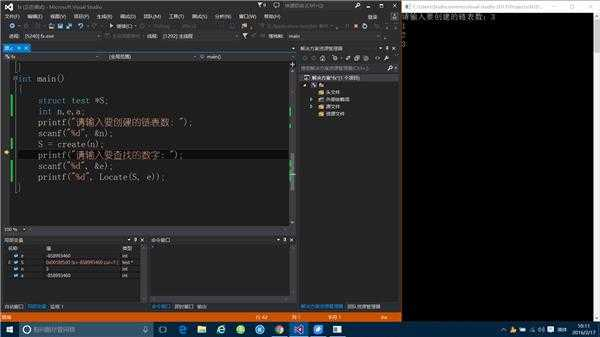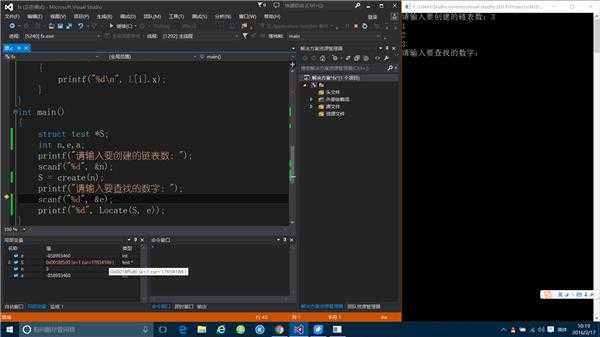﻿ printf会改变地址的值吗？ | Code Bye

# printf会改变地址的值吗？

5年前 (2016-01-30) 609次浏览
```#include<stdio.h>
struct test
{
int x;
int cur;
};
struct test *create(int n)
{
struct test L;
int i;
L.cur = 1;
for (i = 1; i <=n; i++)
{
scanf("%d", &L[i].x);
L[i].cur = i + 1;
}
return L;
}
int Locate(struct test *L,int e)
{
int i;
i = L.cur;
while (i&&L[i].x != e)
i = L[i].cur;
return i;
}
void print(struct test *L,int n)
{
int i;
for (i = 1; i <= n; i++)
{
printf("%d\n", L[i].x);
}
}
int main()
{
struct test *S;
int n,e,a;
printf("请输入要创建的链表数：");
scanf("%d", &n);
S = create(n);
printf("请输入要查找的数字：");
scanf("%d", &e);
printf("%d", Locate(S, e));
}````struct test L;`

`struct test ×L = (struct test *)malloc(sizeof(struct test)*n)`

VC调试时按Alt+8、Alt+7、Alt+6和Alt+5,打开汇编窗口、堆栈窗口、内存窗口和寄存器窗口看每句C对应的汇编、单步执行并观察相应堆栈、内存和寄存器变化，这样过一遍不就啥都明白了吗。

CodeBye 版权所有丨如未注明 , 均为原创丨本网站采用BY-NC-SA协议进行授权 , 转载请注明printf会改变地址的值吗？

[1034331897@qq.com]